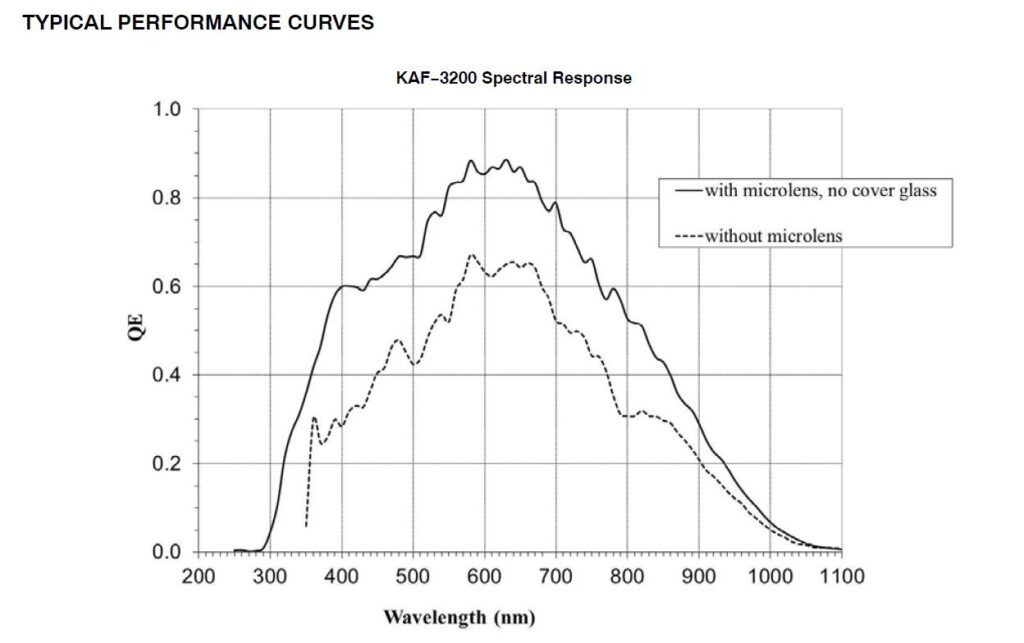### Test report on our new Baader dielectric Bessel filters (prepared by Gianni Galli)

First, I obtained the transmission curves of the filters from the OD data I find in your website. To transform the OD data in curves, I used the following equation:

Transmission = 1/10^OD.

In this way, I was able to get the following curves for U, B, V, R and I filters:

So now I can compare the transmission curve of your filters with the Bessell standard UBVRI filter curves.

My opinion is that your U,V,R filters are well aligned to the standard, while B and I filters show some differences.

B filter shows the so-called “read-leak”, a transmission peak at about 1200 nm: this is a well-known problem in AAVSO and in ANS Collaboration. The first version of Astrodon B filter showed this characteristic: Henden (at that time AAVSO President) asked to Astrodon to modify the B filter and the last version of this one was correct. The “read-leak” can give some problems when you want to measure a very red target, because the CCD not always have zero response over 1000 nm.

It’s difficult to judge the I filter curve, because it shows large transmission over 900 nm, instead I Bessel filter has zero response just over 900 nm.

The V filter shows a minor problem: it is better that at 656,2 nm the transmission is very low (1% or less) because greater transmission can make the measures wrong when the target has a great emission at this wavelength (for example, a nova star). The transmission of your filter is about 5%: this is a percentage higher than Astrodon (0,40%) and Schuler (0,90%) filters while the Optec one is slightly inferior (4,00%).

The Baader filters have shown me the same reflection problem I saw with Astrodon dielectric filters: the reflection of my focal reducer is very evident. But I was able to find a good solution to this problem.

My Schmidt-Cassegrain has a mirror lock and my CCD is fixed to the telescope, so my astronomic setup cannot move reciprocally: I use the TCF-S Optec focuser and I can focus with great precision and above all in an absolutely repeatable way. When I take CCD images of the target I can note the position of the focus (for example, 3500 counts) and when I take flat field images (through a led panel) I can use the same focus position, so the distance between filter and focal reducer in the target images is the same than in the flat ones. In this way, the reflection in my target images and in the flat ones is almost the same and I am able to eliminate it almost completely with the usual calibration technique (I usually use Maxim DL to calibrate my images). The calibrated image shows a good uniformity, with a percentage difference between the brighter zone and the darker one (respect to the average measure of the field) between 1% and 4%, so I am satisfied with this result.

Finally, I measured the transforms of B, V and R filters (the filters I want to use in my photometric activity) following the instructions given by Brian D. Warner in his book “A pratical guide to lightcurve photometry and analysis”.

I observed with the filters above mentioned the Henden field of V371 Per and I measured the transforms of B, V, R filters and the Hidden transforms of (B-V) and (V-R) color index  through Canopus software.

The results are the following:

 With reference to (B-V) color index: With reference to (V-R) color index: T(B-V) = +1,125 +/- 0,030 T(V-R) = +1,170 +/- 0,020 T(B) = +0,183 +/- 0,027 T(B) = +0,367 +/- 0,030 T(V) = +0,066 +/- 0,021 T(V) = +0,131 +/- 0,021 T(R) = -0,006 +/- 0,024 T(R) = -0,024 +/- 0,024

The transforms of V and R filters are good, while the B transform is fairly good.

Some years ago, using a C11, a SBIG ST8XME and Astrodon B, V, R filters, I measured the following transforms:

 With reference to (B-V) color index: With reference to (V-R) color index: T(B-V) = 1,085 +/- 0,012 T(V) = -0,009 +/-0,010 T(B) = 0,072 +/- 0,014 T(R) = -0,031 +/- 0,008 T(V) = -0,009 +/- 0,007 T(V-R) = 1,034 +/-0,009

In the Baader filters tests I used:

Celestron C14 + focal reducer Optec 0,50x with a focal length 2.050 mm (focal ratio 5,76x), CCD Moravian G2-3200 with KAF 3200. The graph of KAF3200ME Quantum Efficiency is:Datasheet from ON Semiconductor

1. 1) Misure di trasmittanza dei filtri fotometrici ANS, by Stefano Moretti ARAR Ravenna
ANS Collaboration, web site http://ans-collaboration.org
2. 2) Lorenzo Franco, private communication

Author: Gianni Galli GiaGa Obs. MPC 203, Pogliano Milanese (MI) Italy